# Divide 5

Divide 288 in the following ratio

3 : 4 : 5

a =  72
b =  96
c =  120

### Step-by-step explanation: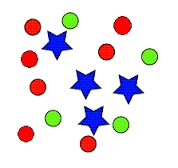Did you find an error or inaccuracy? Feel free to write us. Thank you!Tips to related online calculators
Check out our ratio calculator.
Do you want to perform natural numbers division - find the quotient and remainder?

## Related math problems and questions:

• Moivre 2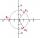Find the cube roots of 125(cos 288° + i sin 288°).
• DivideDivide the number 72 in the ratio 7: 2 and calculate the ratio of the numbers found in this order and write down as decimal.
• 120 nuts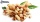Divide 120 nuts in a ratio of 4: 6.
• Divide in ratioLine segment AB 12 cm long divide in a ratio of 5: 3. How long are the individual parts?
• ŽSRCalculate fixed annual personnel costs of operating monorail line 118 km long if every 5 km is a station, which serves three people - one dispatcher and two switchmen in 4-shift operation. Consider the average salary of the employee 885 €.
• TogetherThe three friends divided the balls in a ratio of 6: 5: 4. Some two of them got a total of 126 balls. How many balls were there together?
• Reminder and quotientThere are given the number C = 281, D = 201. Find the highest natural number S so that the C:S, D:S are with the remainder of 1,
• Three numbers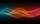The sum of the three numbers is 287. These numbers are in a ratio of 3: 7: 1/4. Find these numbers.
• The balls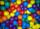You have 108 red and 180 green balls. You have to be grouped into the bags so that the ratio of red to green in each bag was the same. What smallest number of balls may be in one bag?
• Exhibition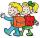The teacher paid 280 Kč for 4.A students for admission to the exhibition. How many students were at the exhibition?
• In theIn the national park, the ratio of the wooded area to grassland is 4: 1. The total area is 385km2. What area is wooded?
• Pension or fraud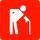Imagine that your entire working life working honestly and pay taxes and to Social Insurance (in Slovakia). You have a gross wage 900 Euros, you pay social insurance (you and your employer) monthly € 288 during 41 years and your retirement is 405 Eur
• RemaindersIt is given a set of numbers { 170; 244; 299; 333; 351; 391; 423; 644 }. Divide this numbers by number 66 and determine set of remainders. As result write sum of this remainders.
• Chicks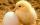How many chicks were hatched from 4500 eggs, when an average of 100 eggs hatched 87 chicks?
• Percentages above 100%What is 122% of 185? What is the meaning of percentages above 100%?
• Probably memberLook at the series 2,6,25,96,285, ? What number should come next?
• FortyForty five of the 80 students were girls. What is the ratio of girls to boys?